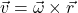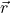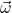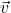## Consider a rotating object. On that object, select a single point that rotates. How does the angular velocity vector of that point compare t

Question

Consider a rotating object. On that object, select a single point that rotates. How does the angular velocity vector of that point compare to the linear velocity vector at that point? a. perpendicular b. parallel c. neither parallel or perpendicular d. Depends on the rotation

in progress 0
2 weeks 2021-09-02T06:05:02+00:00 1 Answers 0 views 0Whereis perpendicular to the rotation axis andis parallel to the same axis. By definition of cross product,is a vector which is perpendicular to both vectors. Therefore, linear velocity vector is perpendicular to angular velocity vector. The correct answer is A.